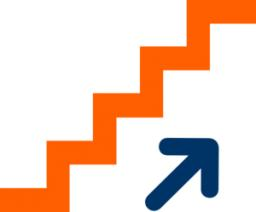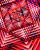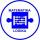# AP - simple

Find the first ten members of the sequence if a11 = 132, d = 3.

a10 =  129
a9 =  126
a8 =  123
a7 =  120
a6 =  117
a5 =  114
a4 =  111
a3 =  108
a2 =  105
a1 =  102

### Step-by-step explanation:Did you find an error or inaccuracy? Feel free to write us. Thank you!## Related math problems and questions:

• Sequence 3Write the first 5 members of an arithmetic sequence: a4=-35, a11=-105.
• Sequence 2Write the first 5 members of an arithmetic sequence a11=-14, d=-1
• AP - simpleDetermine the first nine elements of sequence if a10 = -1 and d = 4
• SequenceWrite the first 7 members of an arithmetic sequence: a1=-3, d=6.
• Sequences AP + GPThe three numbers that make up the arithmetic sequence have the sum of 30. If we subtract from the first 5, from the second 4 and keep the third, we get the geometric sequence. Find AP and GP members.
• GP - sequenceThe first three terms of a geometric sequence are as follows 10, 30. 90, find the next two terms of this sequence.
• AS - sequenceWhat are the first ten members of the sequence if a11=22, d=2.
• SequenceBetween numbers 1 and 53 insert n members of the arithmetic sequence that its sum is 702.
• AP 6Calculate the first five items of an arithmetic sequence if it is given: a2 – a3 + a5 = 20 a1 + a6 = 38
• Sum of membersWhat is the sum of the first two members of the aritmetic progression if d = -4.3 and a3 = 7.5?
• Sequence 11What is the nth term of this sequence 1, 1/2, 1/3, 1/4, 1/5?
• AS sequenceIn an arithmetic sequence is given the difference d = -3 and a71 = 455. a) Determine the value of a62 b) Determine the sum of 71 members.
• SequenceWrite the first 6 members of this sequence: a1 = 5 a2 = 7 an+2 = an+1 +2 an
• SequenceIn the arithmetic sequence is a1=-1, d=4. Which member is equal to the number 203?
• The sumThe sum of the first 10 members of the arithmetic sequence is 120. What will be the sum if the difference is reduced by 2?
• Divisible by 5How many three-digit odd numbers divisible by 5, which are in place ten's number 3?
• Geometric sequence 4It is given geometric sequence a3 = 7 and a12 = 3. Calculate s23 (= sum of the first 23 members of the sequence).